### APPENDIX II: DISTRIBUTION OF THE LEAST-SQUARES SUM

We shall define the vector Ziyi /i and the matrix Fijfj(xi) /i.

Note that H = FT . F by Eq. (27),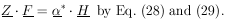(33)

Then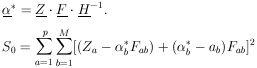(34)

where the unstarredis used for0.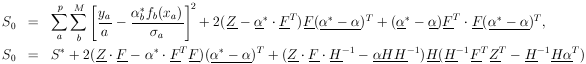using Eq. (34). The second term on the right is zero because of Eq. (33).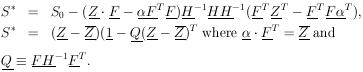(34)

Note that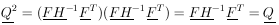If qi is an eigenvalue of Q, it must be equal qi2, an eigenvalue of Q2. Thus qi = 0 or 1. The trace of Q is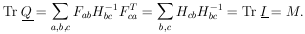Since the trace of a matrix is invariant under a unitary transformation, the trace always equals the sum of the eigenvalues of the matrix. Therefore M of the eigenvalues of Q are one, and (p - M) are zero. Let U be the unitary matrix which diagonalizes Q (and also (1 - Q)). According to Eq. (35),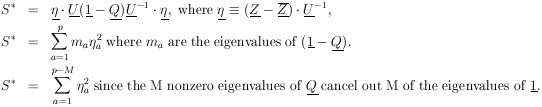Thus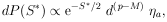where S* is the square of the radius vector in (p - M)-dimensional space. By definition (see Section 16) this is the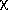2 distribution with (p - M) degrees of freedom.